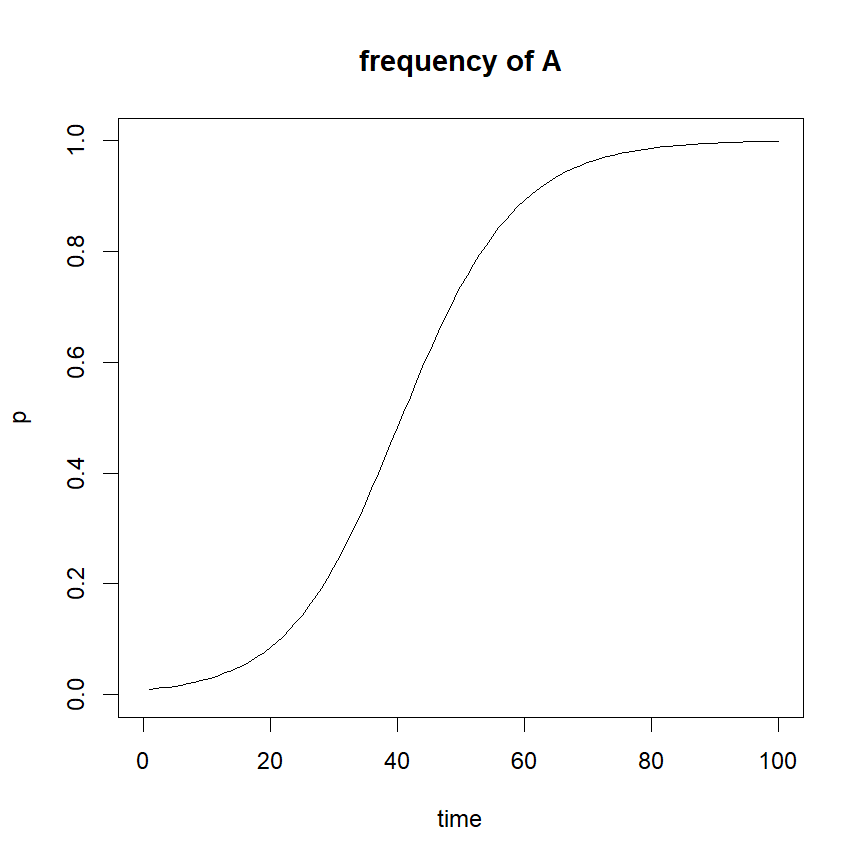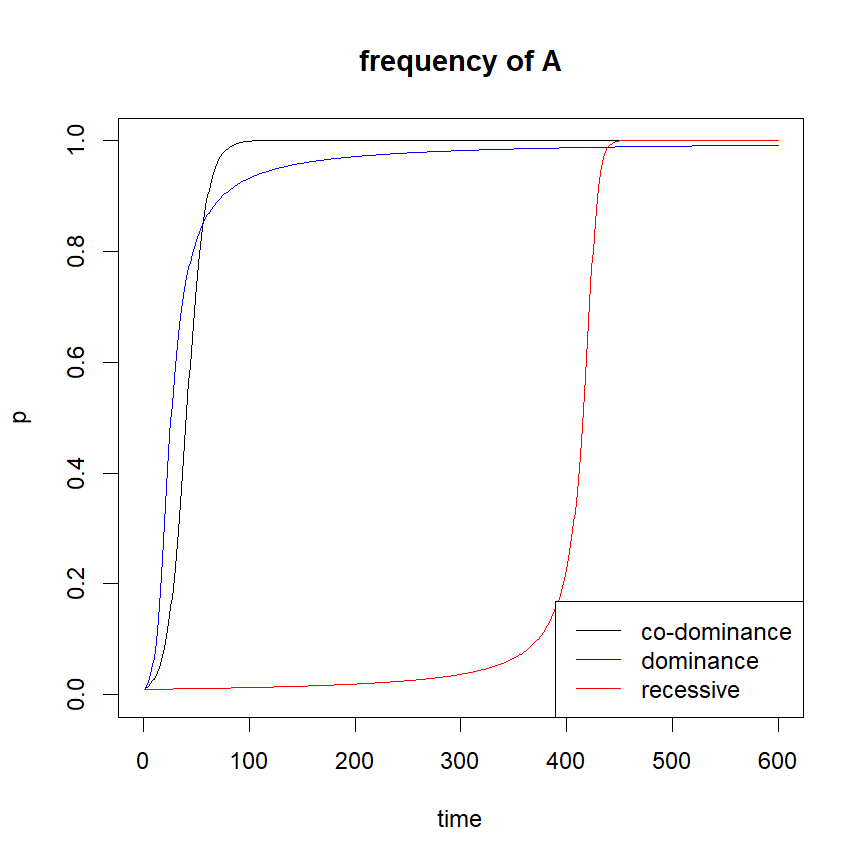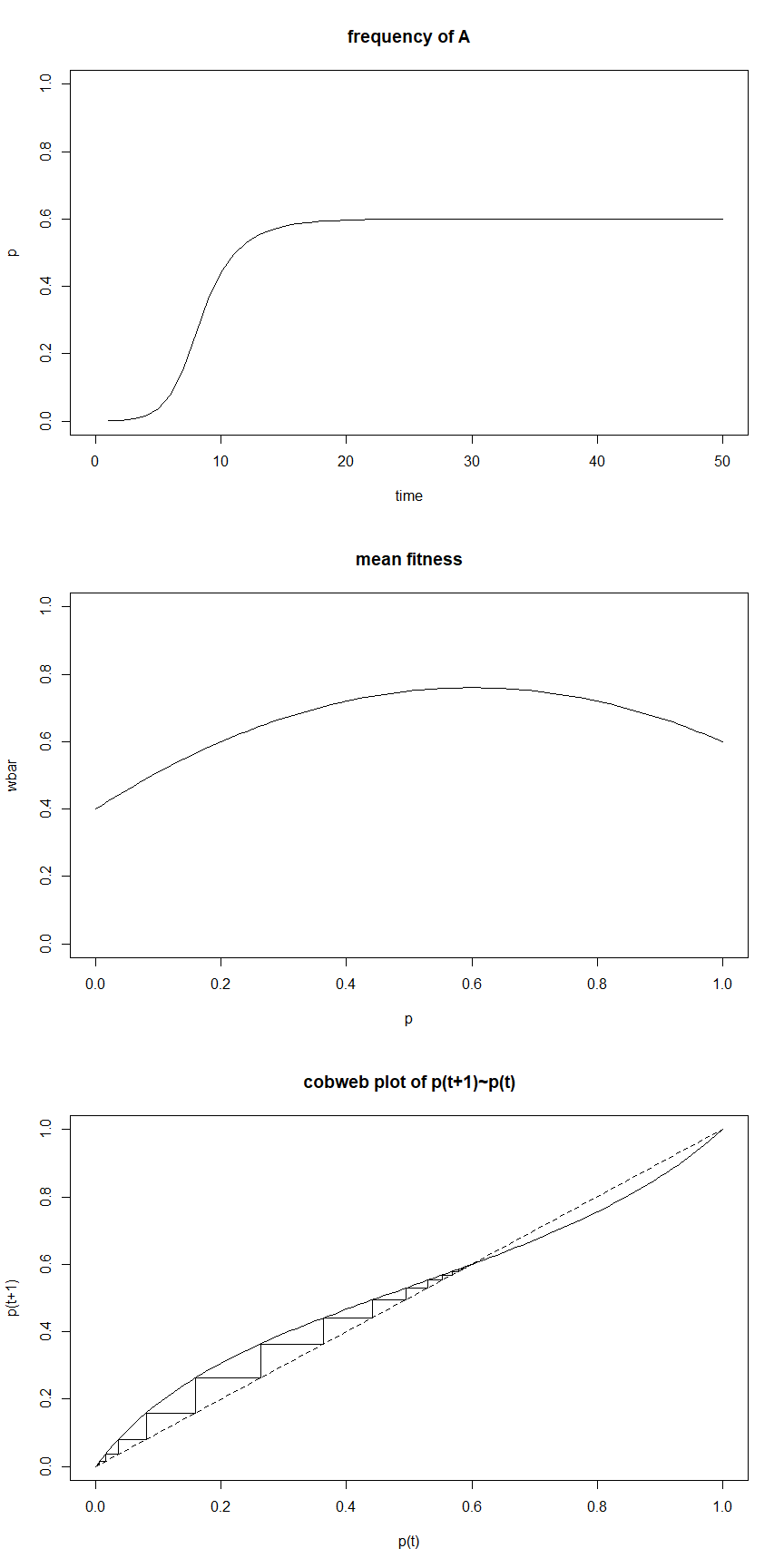## Wednesday, February 14, 2018

### Update to PopGen package for population genetic numerical analysis

Yesterday I updated my little population genetic simulation & numerical analysis package PopGen so that users can overlay the change in allele frequency through time on the same plot.

To install PopGen I would recommend first installing devtools from CRAN after which it can easily be installed directly from GitHub as follows:

``````library(devtools)
install_github("liamrevell/PopGen")
``````

``````library(PopGen)
``````

Now let's use the function `selection` to plot changes in allele frequency through time under a simple biallelic selection model.

The funciont takes as arguments:

``````args(selection)
``````
``````## function (p0 = 0.01, w = c(1, 0.9, 0.8), time = 100, show = "p",
##     pause = 0, ...)
## NULL
``````

which includes the initial frequency of allele A (`p`), the fitnesses of the homozygote (AA), heterozygote (Aa), and homozygote (aa), in that order (`w`), and some other arguments.

First, let's try to run the model under its default conditions:

``````selection()
``````We can modify the argument values of the function. The default conditions show co-dominance for fitness. Let's run a co-dominance, recessive, and dominance for fitness models as follows:

``````## co-dominance for fitness
selection(time=600)
## dominance for fitness
## recessive for fitness
legend(x="bottomright",legend=c("co-dominance","dominance","recessive"),
col=c("black","blue","red"),lty=1)
``````Neat.

The function `selection` can also show other things - such as the fitness surface, and δp as a function of p. Here's what I mean, in this case using a severe overdominance model:

``````par(mfrow=c(3,1))
w<-c(0.6,1,0.4)
selection(p0=0.001,w=w,time=50)
selection(w=w,show="surface")
selection(p0=0.001,w=w,show="cobweb",time=50)
title(main="cobweb plot of p(t+1)~p(t)")
``````